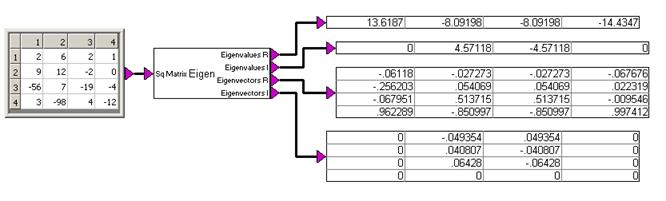## eigenvalues

Block Category: Matrix Operation

Description: The eigenvalues block computes the eigenvalues eigenvectors of a matrix.

The eigenvalues block accepts one square real matrix. It outputs four values: the top two values represent the real and imaginary parts of matrix eigenvalues, and the bottom two values represent the real and imaginary parts of the corresponding right eigenvectors.

#### Example